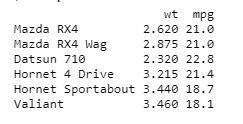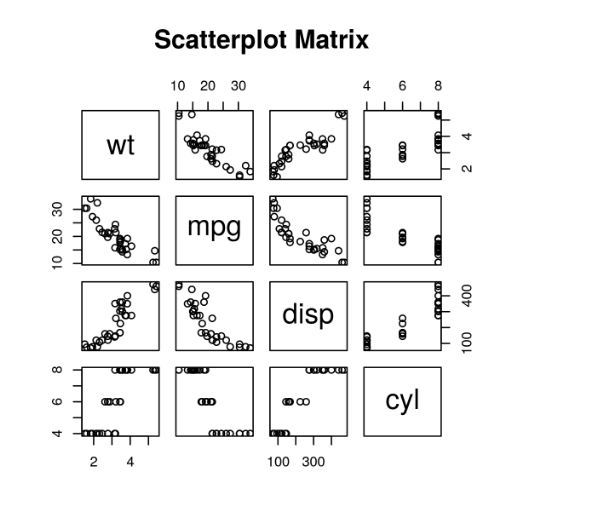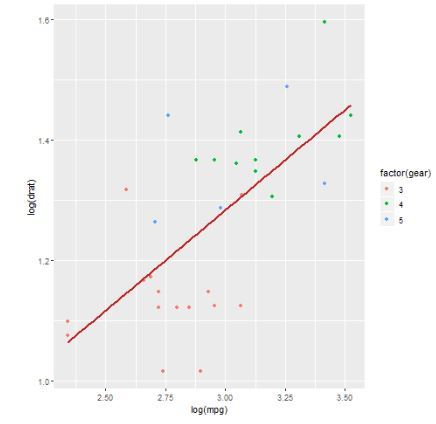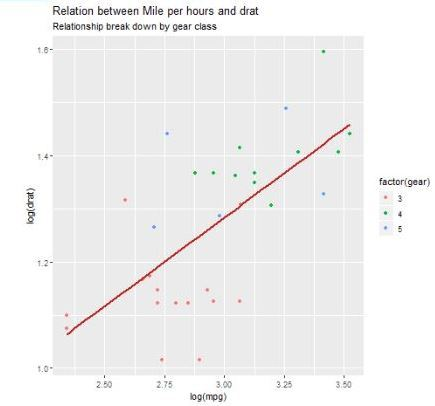# Scatter plots in R Language

A scatter plot is a set of dotted points to represent individual pieces of data in the horizontal and vertical axis. A graph in which the values of two variables are plotted along X-axis and Y-axis, the pattern of the resulting points reveals a correlation between them.

The simple scatterplot is created using the plot() function.

Syntax:

`plot(x, y, main, xlab, ylab, xlim, ylim, axes)`

Parameters:

• x: This parameter sets the horizontal coordinates.
• y: This parameter sets the vertical coordinates.
• xlab: This parameter is the label for horizontal axis.
• ylab: This parameter is the label for vertical axis.
• main: This parameter main is the title of the chart.
• xlim: This parameter is used for plotting values of x.
• ylim: This parameter is used for plotting values of y.
• axes: This parameter indicates whether both axes should be drawn on the plot.

#### Simple Scatterplot Chart

Approach: In order to create Scatterplot Chart:

1. We use the data set “mtcars”.
2. Use the columns “wt” and “mpg” in mtcars.

Example:

 `input` `<``-` `mtcars[, c(``'wt'``, ``'mpg'``)] ` `print``(head(``input``)) `

Output:#### Creating a Scatterplot Graph

Approach: In order to create Scatterplot graph:

1. We are using the required parameters to plot the graph.
2. In this ‘xlab’ describes the X-axis and ‘ylab’ describes the Y-axis.

Example:

 `# Get the input values. ` `input` `<``-` `mtcars[, c(``'wt'``, ``'mpg'``)] ` `  `  `# Plot the chart for cars with  ` `# weight between 1.5 to 4 and  ` `# mileage between 10 and 25. ` `plot(x ``=` `input``\$wt, y ``=` `input``\$mpg, ` `     ``xlab ``=` `"Weight"``, ` `     ``ylab ``=` `"Milage"``, ` `     ``xlim ``=` `c(``1.5``, ``4``), ` `     ``ylim ``=` `c(``10``, ``25``),          ` `     ``main ``=` `"Weight vs Milage"` `) ` `     `

Output:#### Scatterplot Matrices

When we have two or more variables and we want to correlate between one variable and others so we use a scatterplot matrix.

pairs() function is used to create matrices of scatterplots.

Syntax:

`pairs(formula, data)`

Parameters:

• formula: This parameter represents the series of variables used in pairs.
• data: This parameter represents the data set from which the variables will be taken.

Example:

 `# Plot the matrices between 4 variables giving 12 plots. ` ` `  `# One variable with 3 others and total 4 variables. ` `pairs(~wt ``+` `mpg ``+` `disp ``+` `cyl, data ``=` `mtcars, ` `       ``main ``=` `"Scatterplot Matrix"``) `

Output:#### Scatterplot with fitted values

Approach: In order to create Scatterplot Chart:

1. We are using the ggplot2 package provides ggplot() and geom_point() function for creating a scatterplot.
2. Also we are using the columns “wt” and “mpg” in mtcars.

Example:

 `# Loading ggplot2 package   ` `library(ggplot2)   ` `   `  `# Creating scatterplot with fitted values.   ` `# An additional function stst_smooth  ` `# is used for linear regression.   ` `ggplot(mtcars, aes(x ``=` `log(mpg), y ``=` `log(drat))) ``+`  `           ``geom_point(aes(color ``=` `factor(gear))) ``+`  `           ``stat_smooth(method ``=` `"lm"``, ` `           ``col ``=` `"#C42126"``, se ``=` `FALSE, size ``=` `1` `)   `

Output:#### Adding title with dynamic name

Approach: To create Scatterplot Chart, Adding a sub-title:

1. We use additional function, In ggplot we add the data set “mtcars” with this adding ‘aes’, ‘geom_point’.
2. Use the Title, Caption, Subtitle.

Example:

 `# Loading ggplot2 package   ` `library(ggplot2)   ` `   `  `# Creating scatterplot with fitted values.   ` `# An additional function stst_smooth  ` `# is used for linear regression.   ` `new_graph<``-``ggplot(mtcars, aes(x ``=` `log(mpg), y ``=` `log(drat))) ``+` `                      ``geom_point(aes(color ``=` `factor(gear))) ``+`  `                      ``stat_smooth(method ``=` `"lm"``, col ``=` `"#C42126"``,  ` `                      ``se ``=` `FALSE, size ``=` `1``)   ` ` `  `# in above example lm is used for linear regression  ` `# and se stands for standard error.   ` `# Adding title with dynamic name   ` `new_graph ``+` `labs(   ` `        ``title ``=` `"Relation between Mile per hours and drat"``,   ` `        ``subtitle ``=` `"Relationship break down by gear class"``,   ` `        ``caption ``=` `"Authors own computation"`  `)  `

Output:My Personal Notes arrow_drop_upIf you like GeeksforGeeks and would like to contribute, you can also write an article using contribute.geeksforgeeks.org or mail your article to contribute@geeksforgeeks.org. See your article appearing on the GeeksforGeeks main page and help other Geeks.

Please Improve this article if you find anything incorrect by clicking on the "Improve Article" button below.

Article Tags :

Be the First to upvote.

Please write to us at contribute@geeksforgeeks.org to report any issue with the above content.